# Introduction to Stochastic CalculusBack in undergraduate days, when I took my first module on financial mathematics, my professor introduced us by that the most important things are the following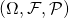This is a probability triple where
1.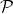is the ‘true’ of physical probability measure
2.is the universe of possible outcomes.
3.is the set of possible events where an event is a subset of.

There is also a filtration, that models the evolution of information through time. For example, if by time, we know that event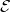has occurs, then. In the case of a finite horizon from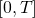, thenA stochastic processis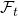-adapted if the value ofis know at timewhen the information represented byis known. Most of the times, we have sufficient information at present.

In the continuous-time model,will be the filtration generated by the stochastic processes (usually a brownian motion,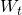), based on the model’s specification.

Next, we review some martingales and brownian motion, alongside with quadratic variation here.

Not readable? Change text.